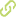# A comprehensive model for piezoceramic actuators: Modelling, validation and application•
• Overview
•
• Identity
•
•
• View All
•

### Abstract

• This paper presents a comprehensive model for piezoceramic actuators (PAs), which accounts for hysteresis, non-linear electric field and dynamic effects. The hysteresis model is based on the widely used general Maxwell slip model, while an enhanced electro-mechanical non-linear model replaces the linear constitutive equations commonly used. Further on, a linear second order model compensates the frequency response of the actuator. Each individual model is fully characterized from experimental data yielded by a specific PA, then incorporated into a comprehensive 'direct' model able to determine the output strain based on the applied input voltage, fully compensating the aforementioned effects, where the term 'direct' represents an electrical-to-mechanical operating path. The 'direct' model was implemented in a Matlab/Simulink environment and successfully validated via experimental results, exhibiting higher accuracy and simplicity than many published models. This simplicity would allow a straightforward inclusion of other behaviour such as creep, ageing, material non-linearity, etc, if such parameters are important for a particular application. Based on the same formulation, two other models are also presented: the first is an 'alternate' model intended to operate within a force-controlled scheme (instead of a displacement/position control), thus able to capture the complex mechanical interactions occurring between a PA and its host structure. The second development is an 'inverse' model, able to operate within an open-loop control scheme, that is, yielding a 'linearized' PA behaviour. The performance of the developed models is demonstrated via a numerical sample case simulated in Matlab/Simulink, consisting of a PA coupled to a simple mechanical system, aimed at shifting the natural frequency of the latter. © 2009 IOP Publishing Ltd.

### Publication date

• November 25, 2009